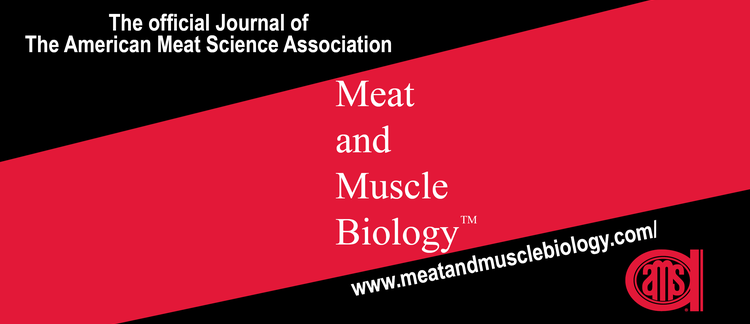Reciprocal Meat Conference Abstracts

### Characterizing Pork Quality of Carcasses with an Average Weight of 119 Kg

Authors: H. Price (University of Illinois) , A. B. Lerner (Kansas State University) , E. A. Rice (Kansas State University) , J. E. Lowell (University of Illinois) , B. N. Harsh (University of Illinois) , K. E. Barkley (University of Illinois) , L. T. Honegger (University of Illinois) , E. Richardson (University of Illinois) , J. C. Woodworth (Kansas State University) , M. D. Tokach (Kansas State University) , S. S. Dritz (Kansas State University) , R. D. Goodband (Kansas State University) , J. M. DeRouchey (Kansas State University) , T. G. O’Quinn (Kansas State University) , M. W. Allerson (Holden Farms) , B. Fields (Pig Improvement Company) , D. A. King (USDA) , T. L. Wheeler (USDA) , S. D. Shackelford (USDA) , A. C. Dilger (University of Illinois) , D. D. Boler (University of Illinois)

•Reciprocal Meat Conference Abstracts

### Characterizing Pork Quality of Carcasses with an Average Weight of 119 Kg

Authors: , , , , , , , , , , , , , , , , , , , ,

Options

## Abstract

ObjectivesBetween 1995 and 2018, average hot carcass weight of U.S. pork carcasses increased from 82 kg to 96 kg, which is an increase of approximately 17%. At current rates, pork carcasses in the U.S. will weigh on average, 105 kg by the year 2030 and over 118 kg by 2050. Although this represents an increase in throughput efficiency due to increases in economy of scale, projecting continued increases in the future raises some concerns. Therefore, the objective was to characterize pork quality of carcasses ranging from 78 to 145 kg with a mean weight of 119 kg.Materials and MethodsCarcass composition, such as hot carcass weight (HCW), back fat depth and loin depth were measured on 666 carcasses. Additionally, loin quality measurements, such as pH, loin instrumental and visual color, and iodine value of clear plate fat were measured on approximately 90% of the total population. Ham quality, 14 d aged loin and chop quality measurements, and loin chop slice shear force (SSF) were evaluated on approximately 30% of the total population. Finally, myosin heavy chain fiber type determination was completed on approximately 50 carcasses selected from carcasses ranging from 97 to 133 kg. The slope of regression lines and coefficients of determination between hot carcass weights and quality traits were calculated using the REG procedure in SAS and considered significantly different from 0 at P ≤ 0.05.ResultsAs HCW increased loin depth (b1 = 0.2496, P < 0.0001), back fat depth (b1 = 0.1374, P < 0.0001), loin weight (b1 = 0.0345, P < 0.0001), chop weight (b1 = 1.6626, P < 0.0001), and ham weight (b1 = 0.1044, P < 0.0001) increased. There was a decrease in estimated lean (b1 = –0.0751, P < 0.0001) and iodine value (b1 = –0.0923, P < 0.0001) as carcass weight increased, however, HCW only accounted for ≤ 24% (R2 = 0.24) of the variation in estimated lean and iodine value. Additionally, there were no significant differences in gluteus medius pH (b1 = 0.0009, P = 0.30) or instrumental lightness (b1 = 0.0301, P = 0.15), redness (b1 = –0.0036, P = 0.73) or yellowness (b1 = 0.0058, P = 0.57) of the ham as carcass weight increased. As carcass weight increased, 1 d loin instrumental yellowness (b*) increased (b1 = 0.0092 P < 0.01), however HCW only explained 1% of the variation in b*. Heavier carcasses were more tender (decreased SSF of chops cooked to 71°C, (b1 = –0.0674, P < 0.0001), although HCW only explained 9% of the variation in SSF. Total cook loss of chops used for SSF determination decreased as HCW increased (b1 = –0.0512, P < 0.0001), and HCW explained 15% (R2 = 0.15) of the variation in total cook loss. There were no significant differences in fiber type percentage, type 1 (b1 = –0.0170, P = 0.81), 2a (b1 = –0.0786, P = 0.23), 2x (b1 = –0.0201, P = 0.80), or 2b (b1 = 0.1224, P = 0.37), or fiber type area, type 1 (b1 = –26.6331, P = 0.22), 2a (b1 = –40.7257, P = 0.07), 2x (b1 = –46.9459, P = 0.25), or 2b (b1 = –26.2537, P = 0.38) as HCW increased.ConclusionDue to the lack of variation explained by HCW (≤ 15%), pork quality traits are not expected to be compromised as HCW continues to increase. The results suggest that increasing HCW to 119 kg did not have detrimental effects on pork quality attributes.

Keywords: color, heavy pigs, pork quality, hot carcass weight, tenderness

How to Cite:

Price, H. & Lerner, A. B. & Rice, E. A. & Lowell, J. E. & Harsh, B. N. & Barkley, K. E. & Honegger, L. T. & Richardson, E. & Woodworth, J. C. & Tokach, M. D. & Dritz, S. S. & Goodband, R. D. & DeRouchey, J. M. & O’Quinn, T. G. & Allerson, M. W. & Fields, B. & King, D. A. & Wheeler, T. L. & Shackelford, S. D. & Dilger, A. C. & Boler, D. D., (2019) “Characterizing Pork Quality of Carcasses with an Average Weight of 119 Kg”, Meat and Muscle Biology 3(2). doi: https://doi.org/10.22175/mmb.10671